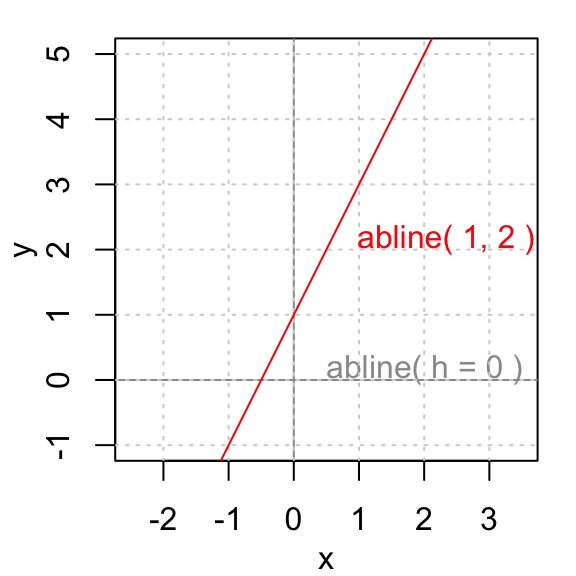# abline R function : An easy way to add straight lines to a plot using R software

The aim of this tutorial is to show you how to add one or more straight lines to a graph using R statistical software. The R function abline() can be used to add vertical, horizontal or regression lines to a graph.A simplified format of the abline() function is :

``abline(a=NULL, b=NULL, h=NULL, v=NULL, ...)``

• a, b : single values specifying the intercept and the slope of the line
• h : the y-value(s) for horizontal line(s)
• v : the x-value(s) for vertical line(s)

The simplified format is :

``abline(v = y)``

It draws a vertical line on the current plot at the specified ‘y’ coordinates.

``````# first example : Add one line
plot(cars)
abline(v=15, col="blue")
# second example : add 2 lines
# change line colors, sizes and types
plot(cars)
abline(v=c(15,20), col=c("blue", "red"), lty=c(1,2), lwd=c(1, 3))
# third example
set.seed(1234); mydata<-rnorm(200)
hist(mydata, col="lightblue")
abline(v = mean(mydata), col="red", lwd=3, lty=2)``````Note that, line types (`lty`) and line width (`lwd`) are explained here.

The simplified format is :

``abline(h = x)``

It draws an horizontal line on the current plot at the specified ‘x’ coordinates.

``````plot(cars)
abline(h=40, col="blue")``````lm() function is used to fit linear models.

``````par(mgp=c(2,1,0), mar=c(3,3,1,1))
# Fit regression line
require(stats)
reg<-lm(dist ~ speed, data = cars)
coeff=coefficients(reg)
# equation of the line :
eq = paste0("y = ", round(coeff,1), "*x ", round(coeff,1))
# plot
plot(cars, main=eq)
abline(reg, col="blue")``````# Infos

This analysis has been performed using R statistical software (ver. 3.1.0).